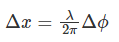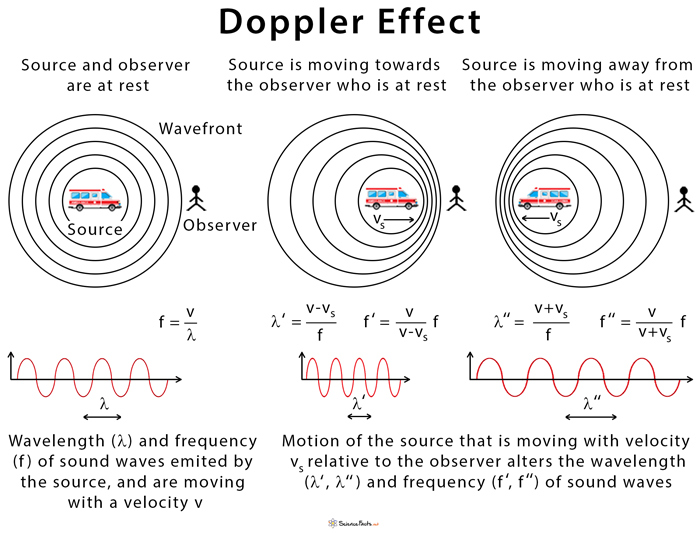# CBSE Class 11 Physics Chapter 15 Revision Notes

Chapter 15: Waves Revision Notes

Waves:

• A wave is a type of disturbance that travels through a material medium as a result of the repeated periodic motion of the medium’s particles about their mean positions without any actual matter transportation.
• Waves have the following characteristics:

(i) The particles of the medium traversed by a wave vibrate only slightly about their mean positions, but they are not permanently displaced in the wave’s propagation direction.

(ii) Along/perpendicular to the wave’s line of travel, each successive particle of the medium performs a motion very similar to its predecessors.

(iii) During wave motion, only energy is transferred, but not a portion of the medium.

• Mechanical or elastic waves, electromagnetic waves, and matter waves are the three main types of waves.

1. Waves of mechanical nature: Only a material medium can produce or propagate mechanical waves. Newton’s laws of motion apply to these waves. Waves on the water’s surface, waves on strings, sound waves, and so on.

2. Electromagnetic Waves are a type of wave that travels through space.

• These are waves that are produced and propagated without the use of a material medium, i.e., they can pass through vacuum and any other material medium.
• Electromagnetic waves include visible light, ultraviolet light, radiowaves, and microwaves, among others.

3. Waves of matter: These waves are associated with moving matter particles such as electrons, protons, and neutrons, among others.

• There are two types of mechanical waves:

(i) Transverse wave motion

(ii) Longitudinal wave motion

• The particles of the medium vibrate at right angles to the wave’s propagation direction in transverse waves.
• Transverse waves include string waves, surface water waves, and electromagnetic waves.
• The disturbance that travels in electromagnetic waves (which include light waves) is caused by the oscillation of electric and magnetic fields at right angles to the wave’s travel direction.
• Particles in the medium vibrate back and forth about their mean position along the energy propagation direction in these types of waves. They’re also known as pressure waves.
• Longitudinal mechanical waves are what sound waves are.

Wavelength:

• The wavelength (λ) is the distance traveled by the disturbance during the time of one vibration by a medium particle.
• A wavelength can also be defined as the distance between two successive crests or troughs in the case of a transverse wave.
• The wavelength (λ) of a longitudinal wave is equal to the distance between the centers of two compressions (or refractions).

Wave Velocity:

• The time rate at which wave motion propagates in a given medium is referred to as wave velocity. It’s not the same as particle velocity.
• The nature of the medium determines the wave velocity.

Wave velocity (υ) = frequency (v) x wavelength (λ)

• Amplitude: The maximum displacement of the medium’s particles from their mean position is the amplitude of a wave.
• Frequency: Frequency is defined as the number of vibrations produced by a particle in one second. It is represented by the letter v. The hertz is the unit of measurement

(Hz) T =1/v

## Factors Influencing Sound Velocity

• The velocity of sound in any gaseous medium is influenced by many factors such as density, pressure, temperature, humidity, wind velocity, and so on.

(i) In a gas, sound velocity is inversely proportional to the square root of the gas’s density.

(ii) If the temperature remains constant, the velocity of sound is unaffected by changes in gas pressure.

(iii) In a gas, sound velocity is proportional to the square root of the absolute temperature.

(iv) The sound velocity in moist air is greater than the sound velocity in dry air.

(v) If the wind is blowing at an angle to the sound propagation direction, the sound velocity is v + w cos θ, where w is the wind velocity.

• Relationship between Path and Phase DifferenceSource

A wave motion can be reflected from both rigid and free boundaries. A traveling wave is reflected with a phase reversal at a rigid boundary or a closed end, but it is reflected without a phase change at an open boundary.

• The Principle of Wave Superposition

The net displacement at a given time is the algebraic sum of the displacements due to each wave at that time when any number of waves meet simultaneously at a point in a medium.

• Stationary waves or standing waves

A new set of waves is formed when two sets of progressive wave trains of the same type (both longitudinal or both transverse) with the same amplitude and time period/frequency/ wavelength traveling at the same speed in opposite directions superimpose. These are referred to as stationary or standing waves.

## Progressive Waves

1. The disturbance continues to spread, passing from particle to particle. Each particle vibrates in the same way as the one before it, but at a different rate.

2. The waves have crests and troughs, which are sine/cosine functions that move with a definite velocity.

3. Each particle has the same amplitude, which it achieves in its own time as the wave progresses.

4. Every particle’s phase changes from 0 to 2 on a continuous basis.

5. No particle can be said to be at rest indefinitely. The particles are momentarily at rest twice during each vibration. At different times, different particles reach this position.

6. All particles have the same maximum velocity, which they achieve one by one as the wave progresses.

7. Energy flows in a regular pattern across all planes in the wave’s propagation direction. In a wave, half of the energy is potential and half is kinetic.

## Stationary Waves

1. The disturbance is stationary, as the wave does not move forward or backward. Each particle has its own set of vibrational properties.

2. In each vibration, the waves resemble a sine/cosine function that shrinks to a straight line twice. It never moves forward.

3. Each particle has a specific amplitude. Some have no amplitude (nodes), while others always have maximum amplitude (antinodes). This is consumed by each participant at the same time.

4. One-half of the waves’ particles have a fixed phase, while the other half of the waves’ particles have the same phase in the opposite direction at the same time.

5. There are particles that are always at rest (nodes), and all other particles have a maximum displacement that they all reach at the same time. In each vibration, these particles are momentarily at rest twice, all at the same time.

6. At the same time, all of the particles achieve their individual assigned velocities based on their positions. Two particles (nodes) in a wave form have constant zero velocities.

7. There is no energy flow in any direction across any plane. Each particle is given its own amount of energy. They all reach their RE. values at the same time, and all energy becomes KB. at a later time.

## Doppler’s Effect

• When there is a relative motion between a source of sound and a listener, the apparent frequencies of sound heard by the listener differ from the actual frequency of sound emitted by the source, according to Doppler’s effect.Source

• Here, v represents the true frequency of the wave emitted by the source,
• v represents the speed of sound through the medium,
• v0 represents the velocity of the observer in relation to the medium, and
• vs represents the velocity of the source in relation to the medium.
• When applying this formula, velocities in the direction OS (i.e., from the observer to the source) are considered positive, while those in the opposite direction are considered negative.
]]>